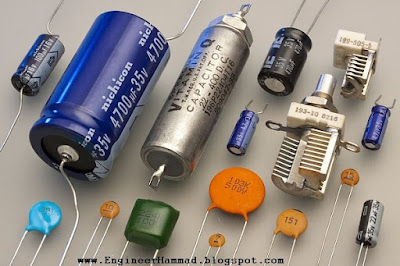## What is Capacitor or capacitor definition:

A capacitor is an electrical passive device which used to store electrical energy. A capacitor is made of two closed conductors / plates, separated by dielectric material.

When  its connected to power source plates accumulates electric charge. Positive and negative charges accumulates on both plates.

Stored electric charge in capacitor is measured as capacitance and the unit to measure capacitance is Farad (F).

### Pictures of capacitors:Capacitors Picture

In the picture shown, these are passive capacitors.

You can see all capacitors have two points to connect with positive and negative charge.

There are different types of capacitors.

### Capacitor symbol:

As shown these are some capacitor symbol.

### what is Capacitance:

Capacitance can be defined as: electric charge divided by voltage is equal to capacitance.
Where:

Q = electric charge (in coulombs)
and
V = Voltage (in volts)

Capacitance Formula: C=Q/V

### Capacitance of a plate capacitor:

Capacitance (C) of plate capacitor is equal to permittivity (ε) times, Area of plate (A) divided by distance between plates (d).

Here,

C = capacitance of capacitor in Farad (F)
ε = permittivity of capacitor's dielectric material in farad/meter (F/m)
A = Area of capacitor plate in square meter (m)
d = Distance between capacitor plates in meter (m)

## Capacitor Construction:

Only Parallel plate capacitors are considered for all practical purposes. There are two conductors or electrodes separated by dielectric material of uniform thickness. Conductors can be any type of material which can easily conduct electricity. Dielectric material must be an insulator - poor conductor.

## Types of Capacitors:

• Variable Capacitor
• Spherical Capacitor
• Electrolytic Capacitor
• Power Capacitor
• Ceramic Capacitor
• Tantalum Capacitor
• Paper Capacitor
• Mica Capacitor

## Jobs in Delta Telecom:

This Job has been Expired you can contact Delta for More Job information.
Delta telecom have opening for Telecom Engineer residing in Saudi Arabia.

## Positions:

• Telecom, Installation Engineer
• Telecom, Implementation Engineer
• FTTH Enginer
• Fiber Optics Engineer
• Generator Tecnician
• Documentation Responsible
• Bss Engineer
• NOC Engineer
• Help Desk Engineer
• Transmission Engineer
• Technicians ( Telecom)
• Riggers
• Purchaser for Telecom Equipment
• Civil Engineer
• Civil Project Supervisor
• Telecom Supervisor